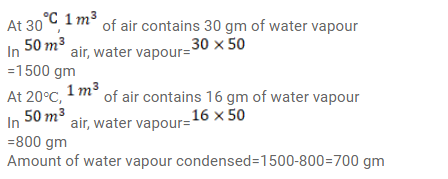# Use R=8.3 J/mol-K wherever required.Question:

Use $\mathrm{R}=8.3 \mathrm{~J} / \mathrm{mol}-\mathrm{K}$ wherever required.

$50 \mathrm{~m}^{3}$ of saturated vapour is cooled down from $30^{\circ} \mathrm{C}$ to $20^{\circ} \mathrm{C}$. Find the mass of the water condensed. The absolute humidity of saturated water vapour is $30 \mathrm{~g} / \mathrm{m}^{3}$ at $30^{\circ} \mathrm{C}$ and $16 \mathrm{~g} / \mathrm{m}^{3}$ at $20^{\circ} \mathrm{C}$.

Solution: